Work, Power & Energy

# Work, Power & Energy - Notes | Study Engineering Mechanics - Mechanical Engineering

 1 Crore+ students have signed up on EduRev. Have you?

Work

Work is performed if an object is forced and the object moves from one location to other location. Work is the consequence of a force that acts at some distance. The distance covered by this body is called it displacement. If the force F, and the displacement d, are in the same direction, then the work W is given by the formula,
W = Fd
The work is the product of the displacement and the force. The force unit is the Newton (N) in SI (international system) units, and the distance or displacement unit is the meter (m). The work unit can therefore be expressed as a Newton-meter. It's also called a Joule, J. The Newton is a composite unit, so a Joule is equivalent to it as well,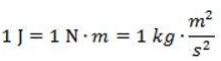The equation W = Fd depends on the same direction as force and displacement. There are many circumstances where, for instance, an individual pushing on a box near the floor is distinct in their direction. Although the resulting direction of the box is forward, a part of the force that the individual applies is aimed down. Only the part of the force along the route of the displacement of the box contributes to the job performed in a situation like this. The work can be found by using the dot product (also known as the scalar product) if we treat force and displacement as vectors.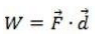W = Fd cos θ
In this formula, θ is the angle between the force and displacement vectors, and F and d are the magnitudes of the vectors.
Whenever work is done against gravity, the amount of work performed is equal to the body's weight product and the vertical distance the body is lifted through.
Work done in lifting body = Weight of Body x Vertical Distance
W = mgh
Where,
W = work Done
M = mass of body
g = acceleration due to gravity
h – height through which the body is lifted

Energy

A body's ability to do work is called energy. Energy is a scalar quantity, i.e. it only has magnitude, but no direction.

1. Potential Energy
The ability to do work in a body because of its situation or setup or we can say a body's energy because of its position or shape change is called potential energy. For example, compressed string energy, gathered water energy at a height, spring energy in a watch, etc.
A body's energy is called gravitational potential energy because of its position above the floor.
A body's energy is called elastic potential energy due to changing its shape and size.

2. Kinetic Energy
An object's kinetic energy (K) is equal to the amount of work needed to accelerate the object from rest to speed, v. This relationship between kinetic energy and work is called the theorem of work-energy. An object's kinetic energy is a scalar value, which means it is not dependent on the direction in which the object moves. The kinetic energy value is always positive or zero. The kinetic energy unit is the same as the Joule (J) unit of work.
An object's kinetic energy can be associated with its mass and velocity with the formula,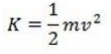If the force is in the same direction as the displacement of the object, this formula for kinetic energy can be discovered from the equation for work. This formula relies on the formula of kinematic.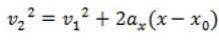The position change (x-x0) is equivalent to the magnitude of the displacement, d. The formula can be rearranged for acceleration, a,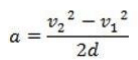Force is equal to the mass of an object multiplied by its acceleration, so the force is,
F = ma
This force formula can be replaced by the work formula,
W = Fd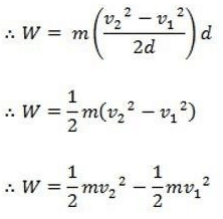The work performed by force on an object is therefore equivalent to the shift in the kinetic energy of the object,
W = K2 - K1
W = ΔK
The Greek uppercase letter ("delta") is used in this formula to mean "change in."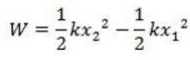Work can be used to comprehend the energy of many objects experiencing forces. The work needed to extend a spring is one instance. If the object's displacement magnitude is marked x, and this represents the displacement away from a position of equilibrium x=0, the force required to pull the object on the spring to a position x is,
F = kx
The constant k in this equation is the spring constant, which is different for every spring.
The spring constant has units Newtons per meter, N/m. The work required to pull the spring from any starting position x1 to a final position x2 is,

Power

Power is a work rate. It is a measure of how fast work is being accomplished. For a quantity of work W performed in time t, the power performed is,
P = W/t
The energy unit is the Watt (W) equivalent to one Joule per second,
1 W = 1 J/s
Power can also be expressed as force F times velocity v. Because work is provided by force times range, W = Fd, and velocity is distance divided by moment, v = d / t, energy is then,
P = W/t
∴ P = (Fd)/t
∴ P = F(d/t)
∴ P = Fv

The document Work, Power & Energy - Notes | Study Engineering Mechanics - Mechanical Engineering is a part of the Mechanical Engineering Course Engineering Mechanics.
All you need of Mechanical Engineering at this link: Mechanical Engineering

## Engineering Mechanics

24 videos|71 docs|58 tests
 Use Code STAYHOME200 and get INR 200 additional OFF

## Engineering Mechanics

24 videos|71 docs|58 tests

Track your progress, build streaks, highlight & save important lessons and more!

,

,

,

,

,

,

,

,

,

,

,

,

,

,

,

,

,

,

,

,

,

,

,

,

;